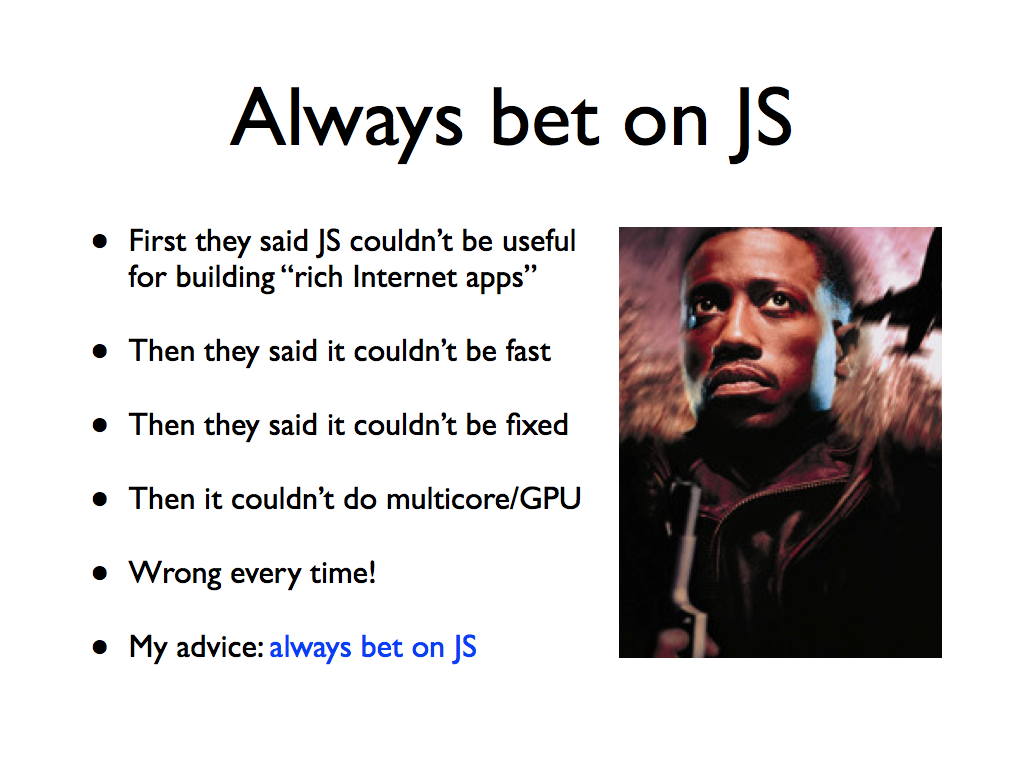# JavaScript at 20

## What was it like to hack JS in 10 days?### Me in 1993, before I created JavaScript in 10 days## Java was the big Web VM

### JavaScript was "little brother"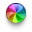### Think back to 1995... what was on TV then?### I had my big break that year...### I'd go to a real library to read Computer Science papers.## "All you had to do was hang around Margaret Jacks Hall."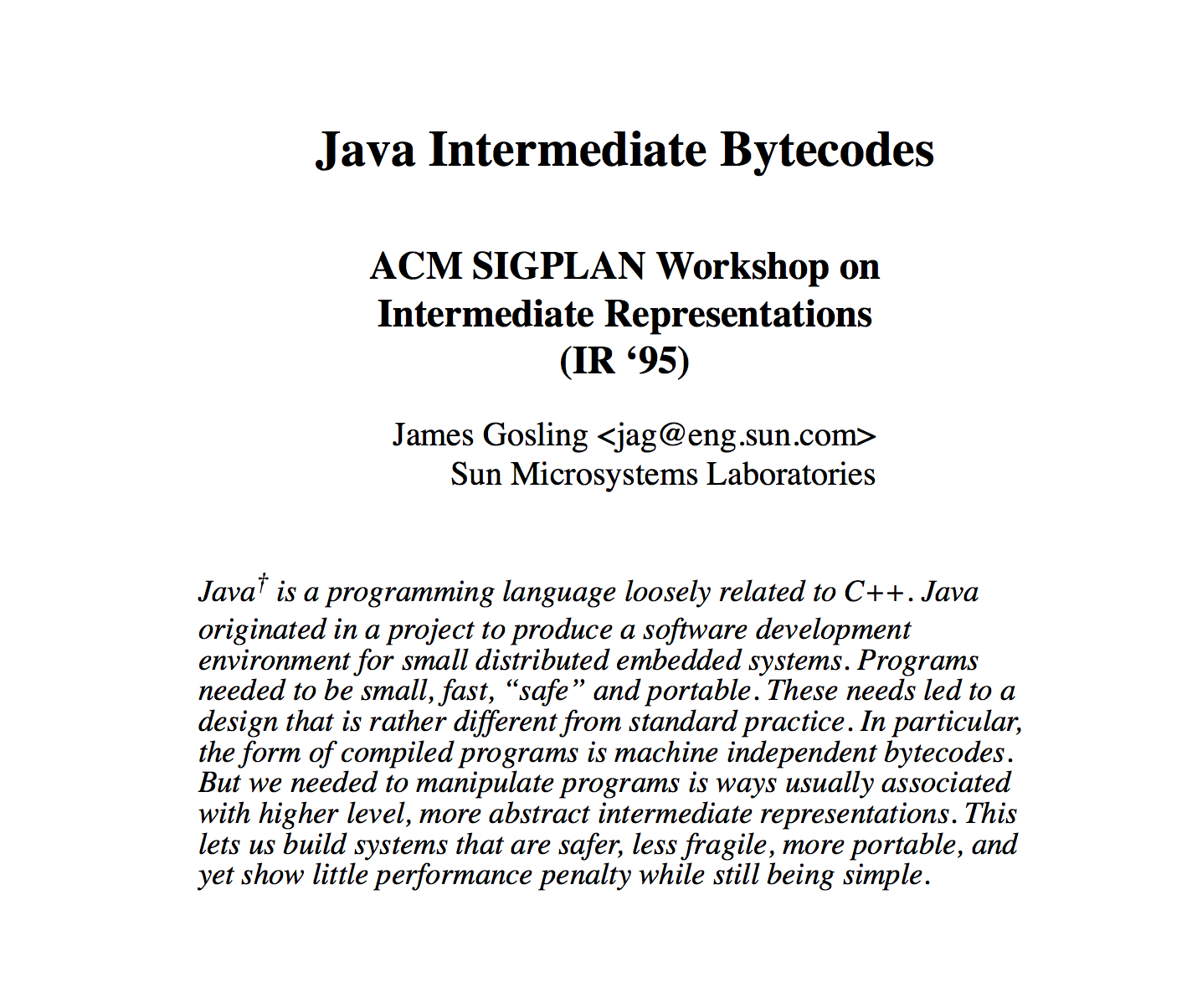### JS is untyped (or dynamically typed)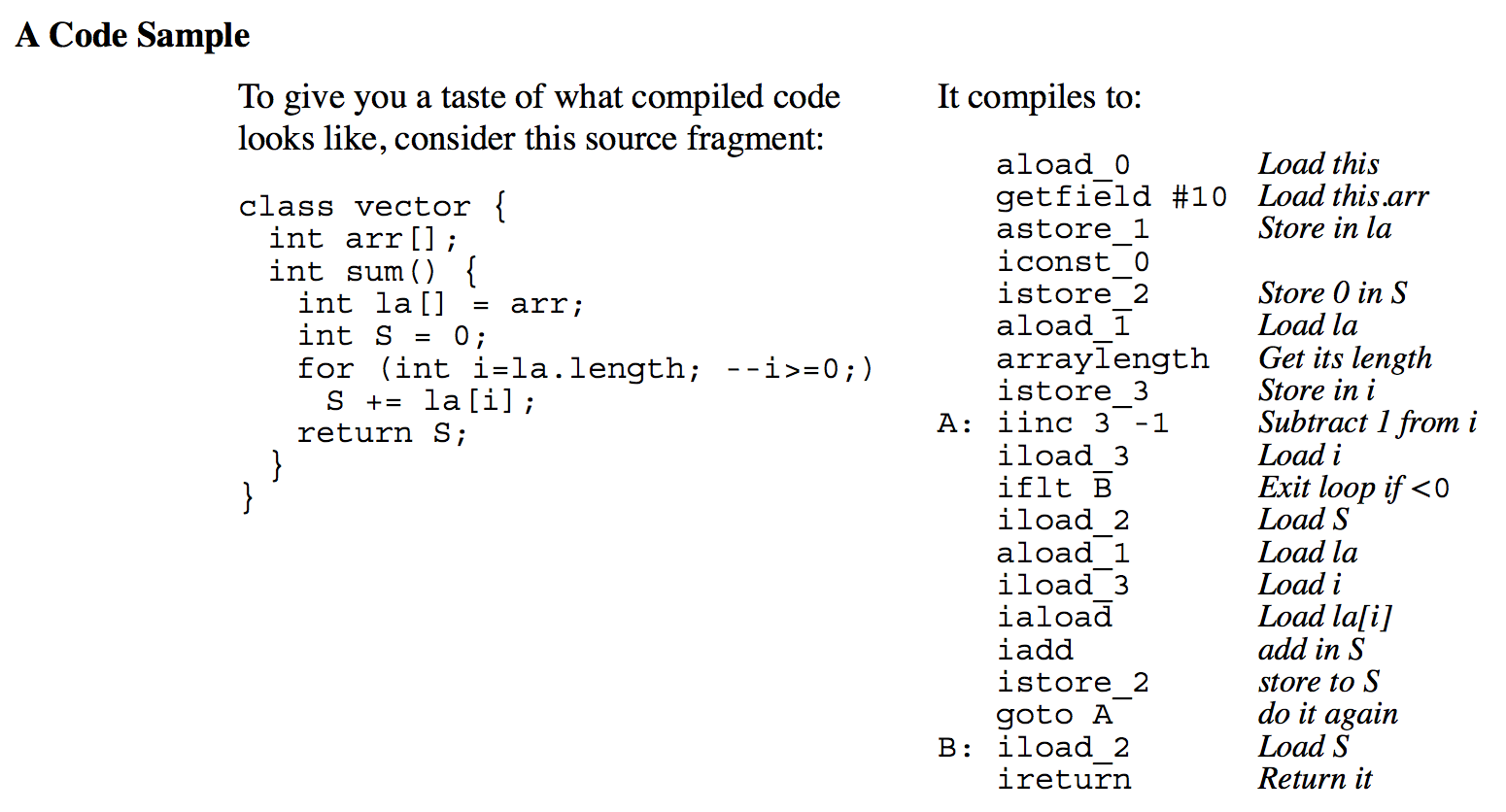### So in 10 days in May 1995, I wrote

• A lexical scanner and parser for early JS
• The parser emitted stack-machine bytecode
• Which ran in a bytecode interpreter
• `Function.prototype.toString` bytecode decompiler
• The standard library was poor
• `Array` was `Object` with `.length` property
• `Date` hand-ported (h/t ksmith@netscape.com) from `java.util.Date`

## Much progress since then

``````class vector {
constructor(n) { this.arr = new Int32Array(n); }
sum() {
let la = this.arr;
let S = 0;
for (let i = la.length|0; (i=(i-1)|0) >= 0;)
S = (S + la[i|0])|0;
return S;
}
}
``````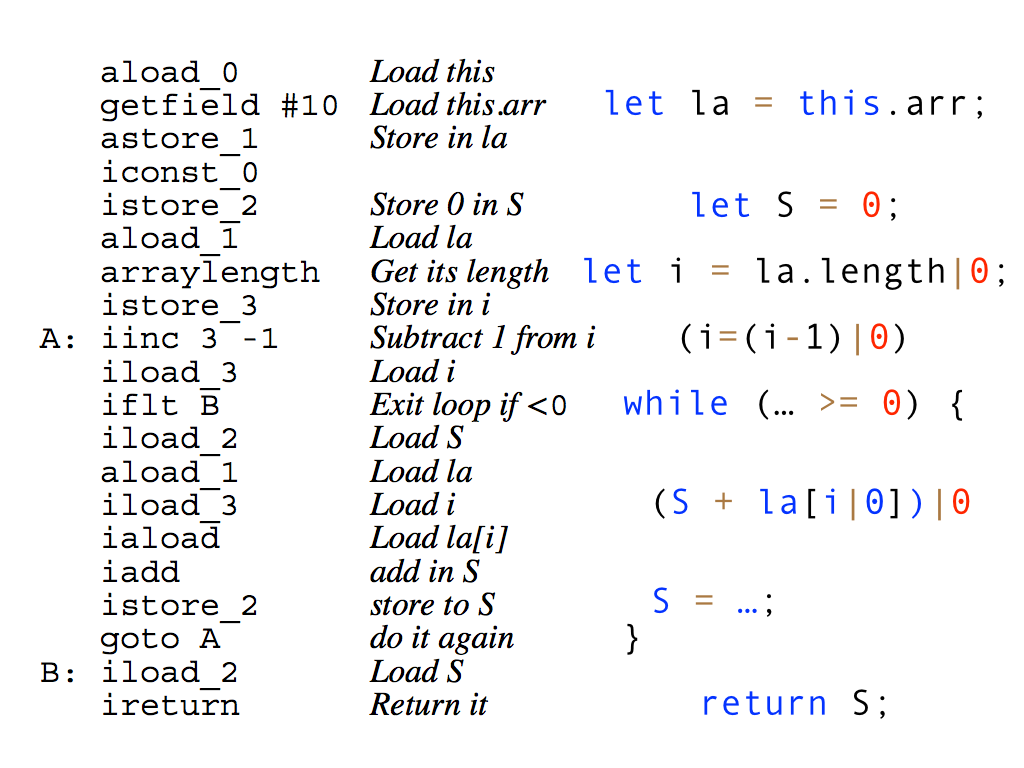## JS VMs compile to the metal

### And the Java VM supports dynamic languages too:## The circle is now complete### Young Jackie Chan## Ecma TC39 on GitHub

### https://github.com/tc39/ecma262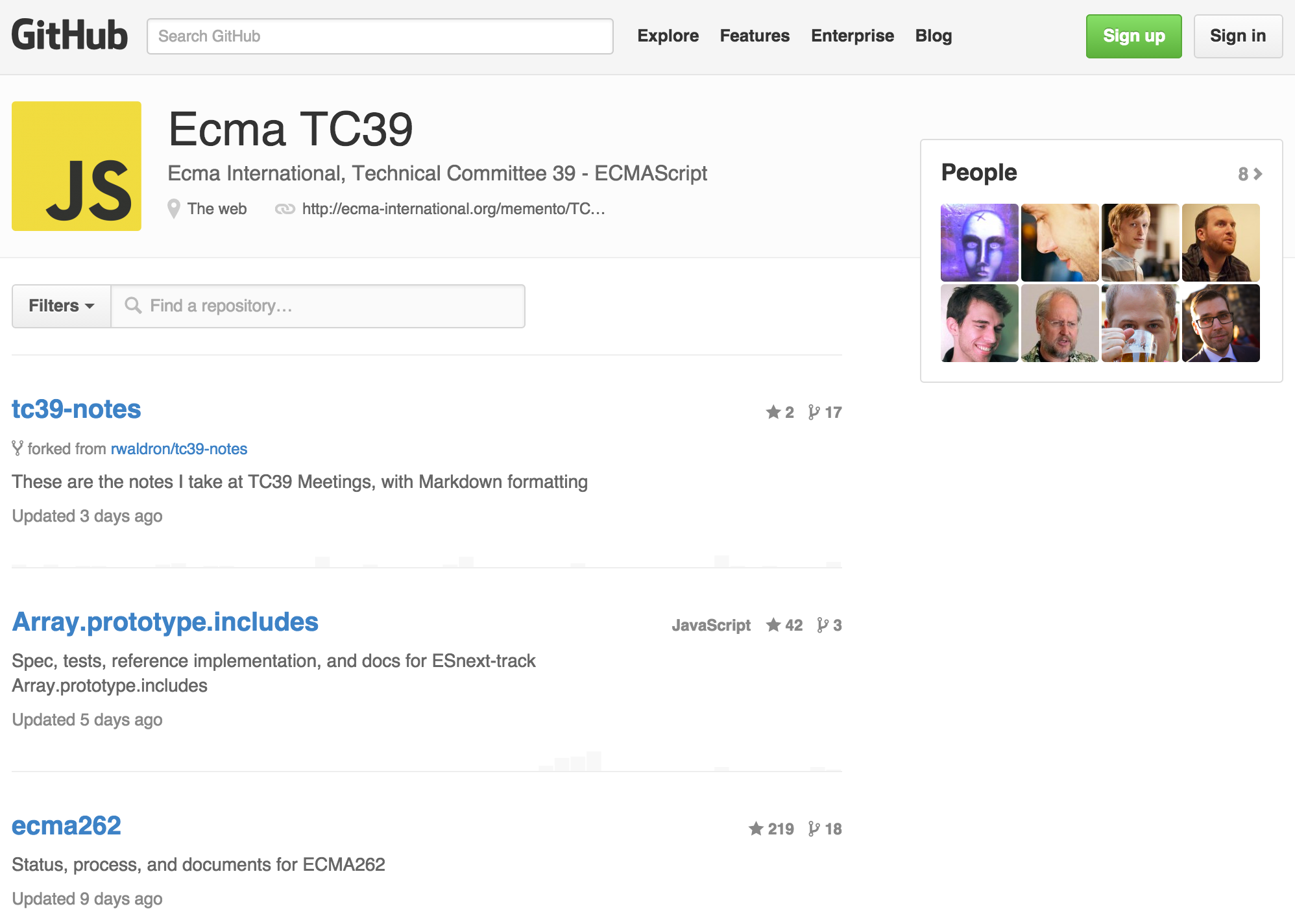## A selective tour of ES6

### `String.prototype.includes(txt, start)`

``````let title = "JS Futures at ModernWeb.tw!";
title.includes("JS")        // true
title.includes("!")         // true
title.includes("XYZ")       // false
title.includes("JS", 3)     // false
https://github.com/tc39/Array.prototype.includes
``````

### `String.prototype.startsWith(txt, start)`

``````let title = "JS Futures at ModernWeb.tw!";
title.startsWith("JS")      // true
title.startsWith("js")      // false
title.startsWith("F")       // false
title.startsWith("F", 3)    // true
``````

### `String.prototype.endsWith(txt, end)`

``````let title = "JS Futures at ModernWeb.tw!";
title.endsWith("!")         // true
title.endsWith(".tw!")   // true
title.endsWith("!", 3)      // false
title.endsWith("Fut", 6)    // true
``````

### `String.prototype.repeat(count)`

``````console.log("x".repeat(3))      // "xxx"
console.log("hello".repeat(2))  // "hellohello"
console.log("abc".repeat(4))    // "abcabcabcabc"
``````

### `String.prototype.trim()`

``````console.log(" x  ".trim())      // "x"
console.log(" \tx".trim())      // "x"
console.log("foo bar\n".trim()) // "foo bar"
``````

### `Template Strings`

• ``````// Verbatim literal string, no backslash escapes
console.log(`In ES6, '\n' is a line feed`);``````
• ``````// Template strings can be multiline
console.log(`In ES6, you can have multiline
strings`);
``````

### `Template String Interpolation`

``````// Interpolate expressions into a template string
var name = "Bob",
time = "today";

console.log(`Hello \${name}, how are you \${time}?`);
``````

### `Tagged Template Strings`

``````function dedent(strings, ...values) {
let result = '';
for (let i = 0; i < strings.length; i++) {
result += strings[i].replace(/\n\s+/g, '\n') + values[i];
}
return result;
}

console.log(dedent `Hello \${name},
How are you \${time}?`);
``````

### Octal and Binary Literals

``````var mode = 0o755;   // Unix permission bits

var bits = 0b101;   // better known as 5
``````

### `Number.isFinite`, `Number.isNaN`

``````console.log(isFinite("25"));        // true
console.log(Number.isFinite("25")); // false

console.log(isNaN("LOL"));          // true!?
console.log(Number.isNaN("LOL"));   // false
``````

## New Math functions

### (h/t Jaydson Gomes)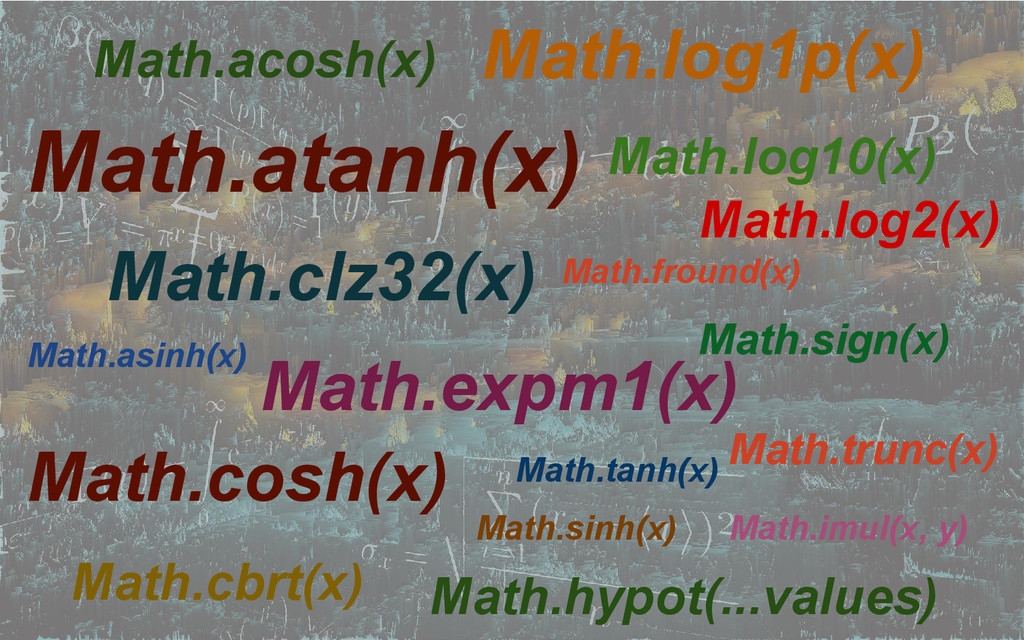### `Array.from(arraylike, mapfun)`

``````let list = document.querySelectorAll('.speaker h2');

console.log(Array.from(list, elem => elem.innerHTML));
``````

### Destructuring (Array Pattern)

``````var m = 3, d = 15, y = 2015;

var [m, d, y] = [3, 15, 2015];

[m, d, y] = getDateTriple();
``````

### Destructuring (Object Pattern)

``````var today = {m: 3, d: 15, y: 2015};

var {m: month, d: day} = today;

console.log(month, day);    // 3 15
``````

### Object Literal Shorthand

``````function longhand(bar, baz) {
return {foo: true, bar: bar, baz: baz};
}

function shorthand(bar, baz) {
return {foo: true, bar, baz};
}
``````

### Block Scope

``````for (var i = 0; i < 3; i++) {
let j = i * i;
console.log(j);   // Works
}
console.log(j);     // Fails

// also const, class, function in block
``````

### Arrow Function Syntax

• Like CoffeeScript's "fat arrow"
• Outer `this` binding
• Not `new`-able
• No `arguments` object
• Always anonymous

### Arrow Function: Expression Body

``````// Single parameter shorthand
let square = x => x * x;
console.log(square(4));     // 16

// 0 or n > 1 parameters case
let empty = () => undefined;
let add = (a, b) => a + b;
``````

### Arrow Function: Block Body

``````let Ackermann = (m, n) => {
if (m == 0) return n + 1;
if (n == 0) return Ackermann(m - 1, 1);
return Ackermann(m - 1, Ackermann(m, n - 1));
}

console.log(Ackermann(2,2));    // 7
console.log(Ackermann(3,3));    // 61
console.log(Ackermann(3,4));    // 125
``````

### Arrows: Great for Callbacks

``````// The classic mistake...
Car.prototype.start = function () {
setTimeout(function () {
this.startDriving();    // Wrong this!
}, 1000);
};

// Much better than using ES5 .bind!
Car.prototype.start = function () {
setTimeout(() => this.startDriving(), 1000);
};
``````

### Classes

``````class Animal {
constructor(name) {
this.name = name;
}

breathe() {
console.log(`\${this.name} is breathing`);
}
}
``````

### Instead of The Prototypal Pattern

``````function Animal(name) {
this.name = name;
}

Animal.prototype.breathe = function() {
console.log(`\${this.name} is breathing`);
}
``````

### Subclassing

``````class Dog extends Animal {
constructor(name) {
super(name);
}

bark() {
console.log(`Woof! \${this.name} is barking`);
}
}
``````

### Subclassing Further

``````class Bulldog extends Dog {
constructor(name) {
super(name);
}

breathe() {
super.breathe();
console.log(`\${this.name} just drooled, too!`);
}
}
``````

## Modules

### Simple Modules

``````// baz.js
let baz = 'baz';

export baz;
// could combine: export let baz = 'baz';

// app.js
import {baz} from "baz.js";
``````

### Default Exports

``````// print.js
export default function print(what) {
return `print module called with \${what}`;
}

// app.js
import print from "print.js";

``````

### Mixing and Matching

``````// foo.js
import {baz} from "./baz.js";
console.log(`from module baz: \${baz}`);

let foo = 'foo';

export default foo;
export let bar = 'bar';

// app.js
import {bar} from "foo.js";
``````

## ES7 async functions

``````function chainAnimationsPromise(elem, animations) {
let ret = null;
let p = currentPromise;
for (let anim of animations) {
p = p.then(function(val) {
ret = val;
return anim(elem);
});
}
return p.catch(e => { /* ignore and keep going */ })
.then(() => { return ret; });
}
``````
``````function chainAnimationsGenerator(elem, animations) {
return spawn(function*() {
let ret = null;
try {
for (let anim of animations) {
ret = yield anim(elem);
}
} catch(e) { /* ignore and keep going */ }
return ret;
});
}
``````
``````async function chainAnimationsAsync(elem, animations) {
let ret = null;
try {
for (let anim of animations) {
ret = await anim(elem);
}
} catch(e) { /* ignore and keep going */ }
return ret;
}
``````

## Demos